# Grouping of Figures

"Grouping of Figures involves segregating a given set of figures into different groups on the basis of some common characteristics."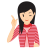Few Important things to Remember

In this section, we have a set of few figures or geometrical shapes which are numbered as 1, 2, 3, 4 and so on. We are required to analyse these figures and classify them into groups consisting of figures having same properties. Grouping of figures can be done on the basis of their shape, orientation, the number of elements and other such characteristics. The best answer is to be selected from a given set of fairly close alternatives.

Directions (Example Nos. 1-2)In the following questions, group the figures into different classes on the basis of their orientation, shape etc.

E.g 1 Group the figures into different classes on the basis of their orientation, shape etc.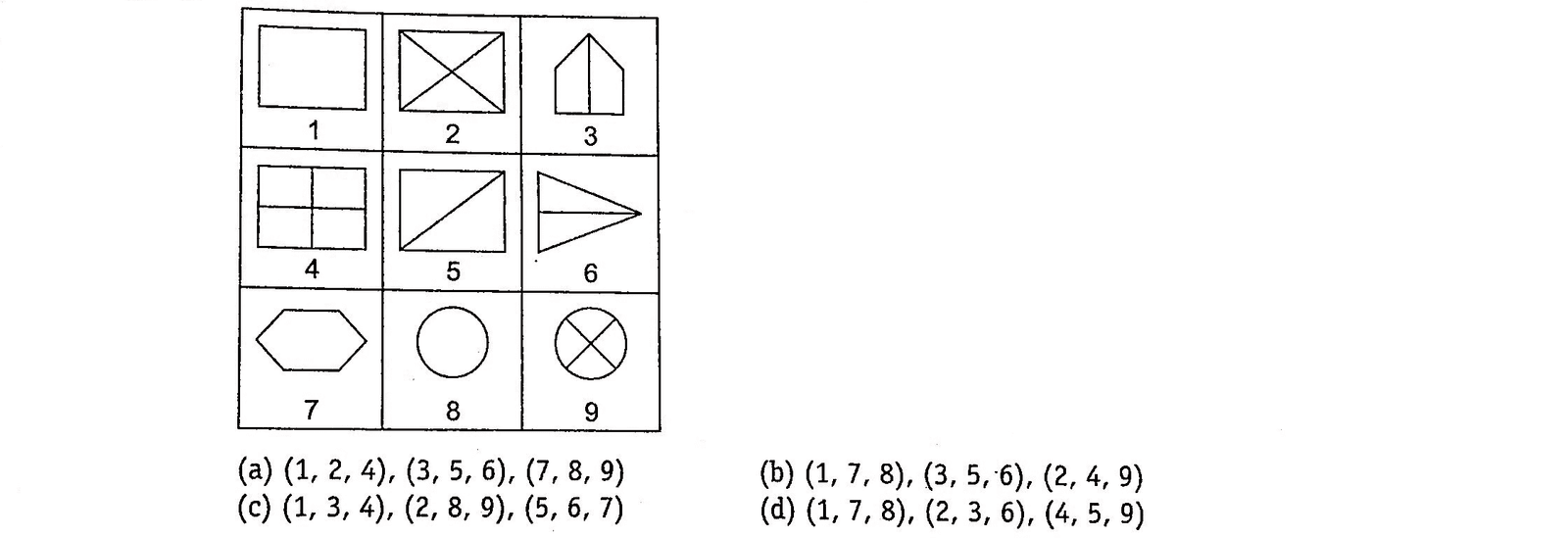Solution: (b)  Clearly, 1,7 and 8 contain a simple geometrical shape. 2, 4 and 9 contain two straight lines dividing the main figure into four parts. 3, 5 and 6 contain one geometrical shape divided into two equal parts by a straight line.

Thus, the given nine figures may be divided into three groups as (1,7,8), (3,5,6) and (2,4,9).

E.g 2 Group the figures into different classes on the basis of their orientation, shape etc.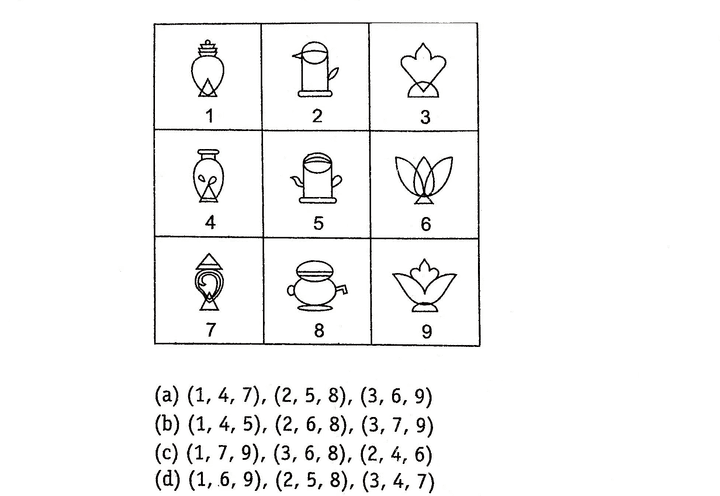Solution: (a)  1, 4 and 7 form a group of figures in which one triangle is present at the base of figure and the figure as a whole looks like a flask.

2, 5 and 8 are kettle shaped figures.

3, 6 and 9 form a group of figures representing floral design.

E.g 3 Which of the following answer figures are similar to class 'A' figures?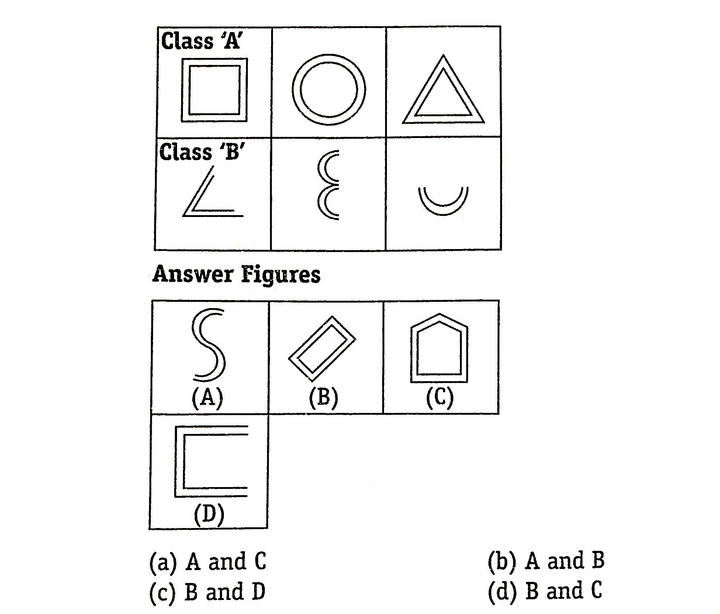Solution: (d)  Each of the three class 'A' figures is composed of two similar and closed geometrical shapes.

Hence, answer figures 'B' and 'C' belong to class 'A'.

E.g 4 Which of the following answer figures are similar ?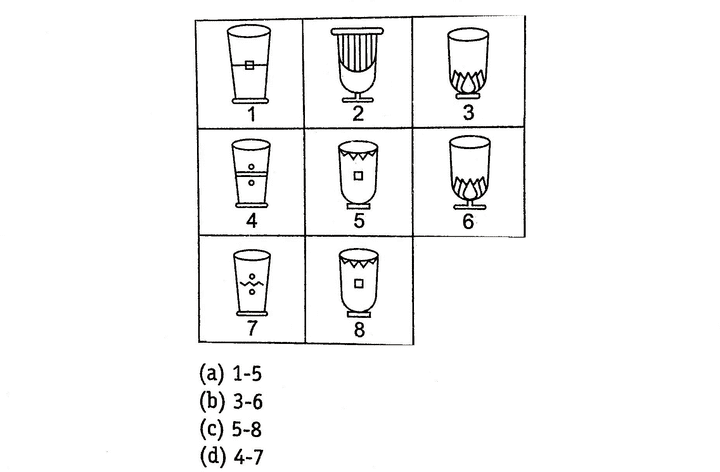Solution: (d)  Clearly, figures 5 and 8 are similar. So, correct option is (c).

LearnFrenzy provides you lots of fully solved Grouping of Figures Questions and Answers with explanation.